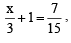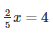# Linear Equations In One Variable - MCQ

## 10 Questions MCQ Test Class 8 Mathematics by VP Classes | Linear Equations In One Variable - MCQ

Description
Attempt Linear Equations In One Variable - MCQ | 10 questions in 10 minutes | Mock test for Class 8 preparation | Free important questions MCQ to study Class 8 Mathematics by VP Classes for Class 8 Exam | Download free PDF with solutions
QUESTION: 1

Solution:
QUESTION: 2

Solution:
QUESTION: 3

### If, then which of the following correct?

Solution:
QUESTION: 4

If 7x + 15 = 50, then which of the following is the root of the equation?

Solution:

7x+15=50
7x=50-15
7x=35
x=35/7
x=5

QUESTION: 5

Ifthen which of the following is the value of x?

Solution:
QUESTION: 6

In the sum of two consecutive numbers is 71 and one of them being x, then which of the following is the other number?

Solution:
QUESTION: 7

Two years ago my age was ‘x’ years 5 years ago my age was?

Solution:
X-3
present age=x+2
before 5 years=x+2-5=x-3
QUESTION: 8

10 years ago I was ‘x’ years old. After 10 years, my age will be:

Solution:
QUESTION: 9

In 15/4 – 7x = 9, x=

Solution:
QUESTION: 10

If ‘x’ is an even number, then which of the following is the next odd number is?

Solution:Use Code STAYHOME200 and get INR 200 additional OFF Use Coupon Code